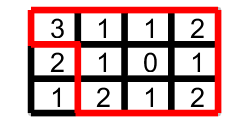# Problem 47463. Slitherlink II: Gimmes

This challenge is to solve Slitherlink pencil puzzles. An essential starter guide is Slitherlink Techniques. An s matrix with values from 0:5 is provided. An s of 5 means this locations edges are not provided and may be from 0:3. The player will be given the s, c, and initial p matrices. The c matrix is clarified for the creation of the solution path of nodes as given in c. The p matrix is a [numel,numel] matrix of c indices where p(x,y)=1 is a possible node connection. p(1,2)=1 as well as example's p(1,5)=1. Additional details of p are provided in the function template. Function template also includes visualization code.
This Slitherlink II: Gimmes is for the cases where s is solved using only the Gimmes from Slitherlink Starting Techniques. The site is missing the Gimme case of adjacent 31 on an edge. Trivial cases may be presented and should be solved prior to processing the Gimmes.
Input: s, matrix of edge counts of the unique solution path; (c,p,bsegs,emap,pmap) are provided but not required
Output: sv, a vector of path nodes where sv(1)=sv(end). These nodes correspond to values in the c matrix example.
Example:
%[1 5 9 13 17 % c matrix [3 1 1 2; % s matrix [1 2 6 7 8 12 16 20 19 18 17 13 9 5 1] % sv
% 2 6 10 14 18 %path nodes 2 1 0 1; %qty edges % sv matrix is vector of nodes generating the
% 3 7 11 15 19 % corners 1 2 1 2] %adjacent % Red Line path
% 4 8 12 16 20] %to path
Related Challenges:### Solution Stats

33.33% Correct | 66.67% Incorrect
Last Solution submitted on Jul 05, 2022

### Community Treasure Hunt

Find the treasures in MATLAB Central and discover how the community can help you!

Start Hunting!